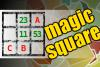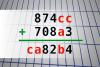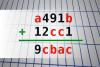BRAIN TEASERSBrain Teasers User Profile

 MAGIC SQUARE: Calculate A*B+C The aim is to place the some numbers from the list (11, 13, 15, 21, 23, 25, 51, 53, 55) into the empty squares and squares marked with A, B an C. Sum of each row and column should be equal. All the numbers of the magic square must be different. Find values for A, B, and C. Solution is A*B+C.Find number abc If 874cc + 708a3 = ca82b4 find number abc. Multiple solutions may exist.Find number abc If a491b + 12cc1 = 9cbac find number abc. Multiple solutions may exist.